# n choose k calculator

Find out how many different ways you can choose k items from n items set without repetition and without order. This number is also called combination number or n choose k or binomial coefficient or simply combinations. See also general combinatorial calculator.

(n)
(k)

# A bit of theory - foundation of combinatorics

## Variations

A variation of the k-th class of n elements is an ordered k-element group formed from a set of n elements. The elements are not repeated and depend on the order of the group's elements (therefore arranged).

The number of variations can be easily calculated using the combinatorial rule of product. For example, if we have the set n = 5 numbers 1,2,3,4,5 and we have to make third-class variations, their V3 (5) = 5 * 4 * 3 = 60.
${V}_{k}\left(n\right)=n\left(n-1\right)\left(n-2\right)\mathrm{.}\mathrm{.}\mathrm{.}\left(n-k+1\right)=\frac{n!}{\left(n-k\right)!}$
n! we call the factorial of the number n, which is the product of the first n natural numbers. The notation with the factorial is only clearer, equivalent. For calculations, it is fully sufficient to use the procedure resulting from the combinatorial rule of product.

## Permutations

The permutation is a synonymous name for a variation of the nth class of n-elements. It is thus any n-element ordered group formed of n-elements. The elements are not repeated and depend on the order of the elements in the group.
$P\left(n\right)=n\left(n-1\right)\left(n-2\right)\mathrm{.}\mathrm{.}\mathrm{.}1=n!$
A typical example is: We have 4 books, and in how many ways can we arrange them side by side on a shelf?

## Variations with repetition

A variation of the k-th class of n elements is an ordered k-element group formed of a set of n elements, wherein the elements can be repeated and depends on their order. A typical example is the formation of numbers from the numbers 2,3,4,5, and finding their number. We calculate their number according to the combinatorial rule of the product:
${V}_{k}^{\mathrm{\prime }}\left(n\right)=n\cdot n\cdot n\cdot n\mathrm{.}\mathrm{.}\mathrm{.}n={n}^{k}$

## Permutations with repeat

A repeating permutation is an arranged k-element group of n-elements, with some elements repeating in a group. Repeating some (or all in a group) reduces the number of such repeating permutations.
${P}_{{k}_{1}{k}_{2}{k}_{3}\mathrm{.}\mathrm{.}\mathrm{.}{k}_{m}}^{\mathrm{\prime }}\left(n\right)=\frac{n!}{{k}_{1}!{k}_{2}!{k}_{3}!\mathrm{.}\mathrm{.}\mathrm{.}{k}_{m}!}$
A typical example is to find out how many seven-digit numbers formed from the numbers 2,2,2, 6,6,6,6.

## Combinations

A combination of a k-th class of n elements is an unordered k-element group formed from a set of n elements. The elements are not repeated, and it does not matter the order of the group's elements. In mathematics, disordered groups are called sets and subsets. Their number is a combination number and is calculated as follows:
${C}_{k}\left(n\right)=\left(\genfrac{}{}{0px}{}{n}{k}\right)=\frac{n!}{k!\left(n-k\right)!}$
A typical example of combinations is that we have 15 students and we have to choose three. How many will there be?

## Combinations with repeat

Here we select k element groups from n elements, regardless of the order, and the elements can be repeated. k is logically greater than n (otherwise, we would get ordinary combinations). Their count is:
${C}_{k}^{\mathrm{\prime }}\left(n\right)=\left(\genfrac{}{}{0px}{}{n+k-1}{k}\right)=\frac{\left(n+k-1\right)!}{k!\left(n-1\right)!}$
Explanation of the formula - the number of combinations with repetition is equal to the number of locations of n − 1 separators on n-1 + k places. A typical example is: we go to the store to buy 6 chocolates. They offer only 3 species. How many options do we have? k = 6, n = 3.

## Foundation of combinatorics in word problems

• First class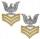The shipment contains 40 items. 36 are first grade, 4 are defective. How many ways can select 5 items, so that it is no more than one defective?
• Probabilities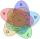If probabilities of A, B and A ∩ B are P (A) = 0.62 P (B) = 0.78 and P (A ∩ B) = 0.26 calculate the following probability (of union. intersect and opposite and its combinations):
• DiceWe throw 10 times a playing dice. What is the probability that the six will fall exactly 4 times?
• AceFrom complete sets of playing cards (32 cards), we pulled out one card. What is the probability of pulling the ace?
• Toys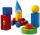3 children pulled 12 different toys from a box. Many ways can be divided toys so that each child had at least one toy?
• Today in schoolThere are 9 girls and 11 boys in the class today. What is the probability that Suzan will go to the board today?
• Birth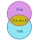Let's assume that the probability of the birth of a boy and a girl in the family is the same. What is the probability that in a family with five children, the youngest and oldest child is a boy?
• Right key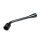The hostel has 4 rooms. The keys to each room are not numbered. Each of the four guests took one key. What is the probability that everyone took the right key?
• The spinner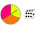The spinner below is spun 12 times. It landed on I 4 times, II 7 times, and III 1 time. What is the difference between the experimental and theoretical probabilities of landing on the II?
• Find the 2Find the term independent of x in the expansion of (4x3+1/2x)8
• White and black ballsThere are 7 white and 3 black balls in an opaque pocket. The balls are the same size. a) Randomly pull out one ball. What is the probability that it will be white? We pull out one ball, see what color it is and return it to the pocket. Then we pull out th
• Combinations 7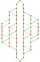A multi-course meal has 3 choices. 3 appetizers, 4 main courses, and 3 desserts. How many different combinations to choose from?
• Three-digit integersHow many three-digit natural numbers exist that do not contain zero and are divisible by five?
• Football league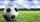In the football league is 16 teams. How many different sequence of results may occur at the end of the competition?
• DicesWe will throw two dice. What is the probability that the ratio between numbers on first and second dice will be 1:2?
• Year 2020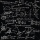The four-digit number divided by 2020 gives a result of 1, **. (Can not be in form 1,*0. ) Write all the options.